## Example Questions

### Example Question #31 : Variables

Define an operation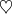on the set of real numbers as follows:

For all real,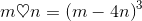How else could this operation be defined?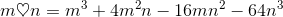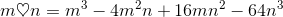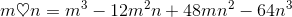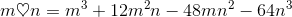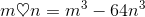Explanation: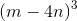, as the cube of a binomial, can be rewritten using the following pattern: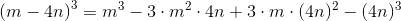Applying the rules of exponents to simplify this: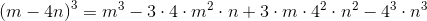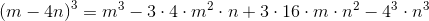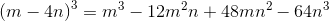Therefore, the correct choice is that, alternatively stated,.

### All SAT Math Resources